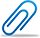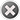Cash-back offer from 1st to 8th February 2023. Get flat 10% cash-back credited to your account for a minimum transaction of \$50. Post Your Question Today!

Question Details Normal
\$ 25.00

# Computer Science Project

Question posted byProblem 1

Consider the system of simultaneous non-linear algebraic equations

3x - y = z

2x^2 - y = 0

where z = −2. Symbolically, use the subs command to substitute the value of z in the first equation, then use the solve command to solve this system of equations. Be sure to print any fractions as fractions. The result of this system will be symbolic, so to print the two solutions as fractions it will be easiest to convert the symbols to character strings using the char command; this will preserve the fractional form, where appropriate.

Hints: 1) The solutions are (x, y) = 2,8 and (x, y) = −  (-1/2, 1/2) Consider how you want to write the equation inside of the solve commands – some approaches can be easier than others. 3) When printing, remember to print the entirety of the string, not just an individual character.

Problem 2

Consider the system of simultaneous ordinary differential equations

dx/dt = 4x - 2y

dy/dt = 3x - y

with initial conditions x(0) = 1 and y(0) = 2. Symbolically solve for x(t) and y(t) with respect to the default variables, and display the results using a combination of the simplify and pretty commands. Before each pretty command, use a print statement to label what the “pretty” equation represents.

Hints: (1) It is difficult to print the label and the result from the pretty operation on the same line; feel free to leave them on separate lines in the Command Window (2) A partial solution is:

x(t) is:

-exp(t)  (exp(t) - 2)

Problem 3

Consider the polar function r = 2 sin (2.7 theta)

Graph this function using the ezpolar command for theta ∈ [0, 40pi]. The command ezpolar has similar syntax to ezplot; if you want to double check it, see the help or doc pages.

Problem 4

The linear velocity of a space vehicle is given as v(t) = 4 cos (3t) + 5 e^(-0.25t)

where t is in seconds and v(t) is in meters/second. From physics, we know that the position x(t) is the integral of the velocity, and that based on this system its units would be meters. Similarly, acceleration a(t) is the derivative of the velocity, with units of meters/sec^2 .

Symbolically, find position x(t) by calculating the indefinite integral of v(t); neglect units when reporting the result of the integral calculation. What is the distance in meters that has been traveled between t = 3 sec and t = 4 sec? You can find this using the Fundamental Theorem of Calculus and substituting the integration bounds into the result of the integral. Recall that the Fundamental Theorem of Calculus states that if F(x) is the antiderivative of f(x) then it follows that [integral from a to b] f(x) dx = F(b) - F(a).

Symbolically, find the acceleration a(t) by calculating the derivative of v(t) ; neglect units when reporting the result of the derivative calculation. What is the acceleration in m/s at t= 4.5 sec?

Hints: (1) To display your symbolic results, consider using the pretty and/or simplify commands; cf. hint 1 of Problem 2. (2) The double command can be used to convert symbols to doubles; the numeric results will not appear as expected, so use this command to correct that.

Problem 5 (**I can email you the csv file, just messege me**)

A technologically un-savvy professor in an undisclosed college at Penn State has hired you to create a program that will do statistical calculations on his final exam scores over 40 years. The final exam scores for all of his class are provided in the file Project2Data.csv, which may be found in the “Data Files” folder on ANGEL; all of the data is already concatenated into a column vector for you. The grading scale as stated in his syllabus is:

Grade         F     D     C   C+   B-   B    B+    A-    A

Minimum    0    60   70   77   80   83   87    90   93

You may assume the lowest grade in the file is a score of 0. Programmatically open the file in MATLAB, saving the data into a variable. For this data set, storing each as (a) separate variable(s) (as applicable), programmatically perform the following tasks:

a) The number of students in the class

b) The highest grade in the class c) Use the find command to determine how many students received each grade (A, A-, B+, etc.), storing the number of each letter grade in a vector. Calculate the wall time for this operation (in seconds) with the tic and toc commands, storing the result as a variable. You may have to use the find command repeatedly, but do not use a loop!

d) Repeat c) (including the wall time calculation), this time using a loop and an if selection structure. Please store the results in different variables than those used in c).

e) For this sample size, what was the performance difference in wall time between the approaches you took in c) and d)? Do you anticipate the performance to change if the class size were to change? Store the text from your responses to these two questions in a variable using the sprintf command. When you do this, make sure you print the times measured on your machine, since we are going to get different values when we run your code on our machines. Hint: Report what you observe, even the result does not match your expectations; as we discussed in class, MATLAB’s loop performance has improved over the years.

f) Use print commands to print the results for items a) through e) to a text file entitled username_project2.txt (example: bjs5332_project2.txt). Format it however you like, but each item must contain a label, (a) value(s), and unit(s) (as appropriate). Do not use any loops to accomplish this task.

g) Using either c) or d) (your choice), print at the end of the same file described in f) the number of students that achieved each of the 9 letter grades. Since you are repeating this process more than 3 times, use a loop. Create a variable that contains character strings that contains each of the letter grades (A, A-, B+, etc.), and call the correct element of this matrix on each pass of the loop; use a cell array to store the labels; while it is possible to use a character array for this task, it is likely more trouble – in this case – than it is worth.

h) Close the text file you created in f).

Note: Between parts f) and g), you should end up printing the same information 3 times. You should verify that the answers are the same each time; if not, you have made 1 or more mistakes.Attachment
Other Related QuestionsMarkT...

### Computer Science and Engineering 2021.03 Sample Final Test

Status : 1 solutiontaka1...

### Computer Science

Status : 1 solutionXBlack

### Principles of Computer Science

Status : 1 solutionmzazi...

### Basic computer skills

#### The benefits of buying study notes from CourseMerits##### Assurance Of Timely Delivery
We value your patience, and to ensure you always receive your homework help within the promised time, our dedicated team of tutors begins their work as soon as the request arrives.##### Best Price In The Market
All the services that are available on our page cost only a nominal amount of money. In fact, the prices are lower than the industry standards. You can always expect value for money from us.